Q-Chem 5.0 User’s Manual

# 10.19 Molecular Junctions

In molecular junctions, molecules bridge two metallic electrodes. The conductance and current-voltage relationship of molecular junctions can be calculated using either Landauer or Non-Equilibrium Green’s Functions (NEGF). In both cases, the Green’s function formulation is employed using a chosen level to describe the electronic structure. See Refs. Datta:2005,DiVentra:2008 for further introduction.

In molecular junctions the current-voltage curve depends on the electron transmission function, which can be calculated using the quantum transport code developed by the Dunietz group (Kent State). The scattering-free approach, (Landauer), provides a zero-bias limit, whilst the non-equilibrium approach, (NEGF), iteratively solves for the junction under the effect of the finite-voltage biased system.

This quantum transport utility is invoked by setting the $rem variable TRANS_ENABLE. TRANS_ENABLE  To invoke the molecular transport code. TYPE:  INTEGER DEFAULT:  0 Do not perform transport calculations (default). OPTIONS:  1 Perform transport calculations.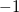Print matrices needed for generating bulk model files. RECOMMENDATION:  None Output is provided in the Q-Chem output file and in the following files (for closed shell system in the spin-restricted framework): • transmission.txt (Transmission function in the requested energy window) • TDOS.txt (Total density of states) • current.txt (I-V plot only for the Landauer level) • FAmat.dat (Hamiltonian matrix for follow up calculations and analysis) • Smat.dat (Overlap matrix for follow up calculations and analysis) • IV-NEGF-all.txt (I-V plot obtained by NEGF method) In the case of unrestricted spin the transmission is calculated for each spin-state as indicated by the A[B] appended to the file names listed above (e.g. transmissionA.txt and transmissionB.txt). (The file name with the letter AB indicates output data including both spin states (e.g. transmissionAB.txt). We note that in the closed-shell spin-restricted case, the transmission.txt corresponds to thespin, where the total transmission due to the spin symmetry is twice the values included in the file. In the NEGF calculation, the above output files are placed in the directories, Vbias1, Vbias2,(the numbers in the directory names are index of bias voltage), where these output files in each directory are of data at the given voltage. T-Chem requires setting parameters in two transport-specific sections in the input file: •$trans_model (molecular model regions)

• $trans_method (Specifies the mode to calculated the electrode self-energies) The$trans_model section provides the number of basis functions in the different regions (molecular model partitioning). There are no default values given for these parameters. The different regions are illustrated in Fig. 10.1 with a six carbon atom chain based bridge used as an example of the Landauer calculation. The partitioning as well as the scheme for the NEGF calculation is illustrated in Fig. 10.2. In these systems the electrodes are represented by a chain of Au atoms. In this example of electrode wires, each single atom represents one layer.

The necessary parameters in the $trans_model section are as follows: • trans_latom: INTEGER, atom index in the$molecule section where the central/bridge region starts (the first atom in junction area).

• trans_ratom: INTEGER, atom index in the $molecule section where the central/bridge region ends (the last atom in junction area). • trans_lgatom: INTEGER, atom index where the repeat unit of the left electrode starts. • trans_rgatom: INTEGER, atom index where the repeat unit of the right electrode ends. See Fig. 10.1 for illustrations of the different regions, and the way the parameters define them. Atoms within numbers trans_lgatom, trans_lgatom+1, .., trans_latom-1 define the repeat unit of the left electrode (similarly for right electrode). Alternatively, the different regions can be provided with the atomic orbital (AO) index as follows: • trans_lbasis: INTEGER, the number of basis functions appearing to the left of the device region (the index of the first AO within the central region). • trans_rbasis: INTEGER, the number of basis functions appearing to the right of the device region right electrode (total number of basis functions minus this number equals last AO that is within the device). • trans_lgbasis: INTEGER, the number of basis functions of the repeat unit of the left electrode. • trans_rgbasis: INTEGER, the number of basis functions of the repeat unit of the right electrode. For the NEGF calculation, trans_lbasis and trans_lgbasis must be the same number as shown in Fig. 10.2 (as for trans_rbasis and trans_rgbasis. Note: The assignment of trans_latom etc. has priority. If trans_latom is specified, then trans_lbasis is ignored. Similarly for trans_latom and trans_lbasis. For the example in Fig. 10.1, if there are a total of 18 atoms and the Au and Ag basis sets each contain 22 basis functions per atom and the repeat unit includes a pair of Au Ag atoms, then the parameters should be given as follows (only the first two columns are required, the rest are included for explanation):  trans_lbasis 88 No. of functions representing left electrode region (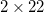for Ag +for Au) trans_rbasis 88 No. of functions representing right electrode (for Ag +for Au) trans_lgbasis 44 Size of the repeating unit of the left electrode (22 for Ag + 22 for Au) trans_rgbasis 44 Size of the repeating unit of the right electrode (22 for Ag + 22 for Au) Or use the atom numbers corresponding to their position in the$molecule section:

 trans_lgatom 3 Third atom is used to define the repeat unit of the left electrode trans_latom 5 Fifth atom is the first atom of the junction trans_ratom 14 Fourteenth atom is the last atom of the junction trans_rgatom 16 Sixteenth atom is used to define the repeat unit of the right electrode

In this example, we have used for the same repeat unit for the left and right electrodes; this symmetry is not required.

Note: The order of atoms in the $molecule section is important and requires to following: Repeating units (left) - Molecular Junction - Repeating units (right) The atoms are provided first by the leftmost repeat unit with the left electrode then proceeds to the next repeat unit up to the surface unit. Next the bridge atoms are provided followed by the right surface unit and the right electrode region. The right electrode region starts with the surface layer and ends with the most distant layer within the bulk. The atoms order within each electrode layer (the repeat units) must be consistent. The atoms order within the bridge region (excluding the electrode repeat unit atoms) is arbitrary. That is, the order of atoms in the molecule section has to adhere to the following: 1. atoms of the leftmost repeat unit 2. atoms of the next repeat unit 3. atoms of the left surface unit device 4. bridge atoms 5. atoms of the right surface unit 6. atoms of the next right electrode unit 7. atoms of the rightmost repeat unit T-Chem allows for complete flexibility in determining the different regions of the electrode models. As a consequence, incorrect setting of regions is not caught by the program and may produce transmission functions that are unphysical (e.g. large values or even negative). Such errors can occur where the cluster model is partitioned (by mistake) within the orbital space of an atom. Regions must always be defined between atomic layers. Each repeat unit atoms should be always provided with the same internal order. Note: At least a single repeat unit of the electrodes should be included in the bridge region. With the Landauer model, if trans_readhs == 0, then at least one layer beyond the bridge region has to be included, an additional layer (total of two or more) is required when trans_method != 0. The necessary parameters in$trans_method section are listed as follows,

trans_mode
 Mode of calculation.

INPUT SECTION: $trans_method TYPE:  INTEGER DEFAULT:  1 Landauer level. OPTIONS:  3 A self-consistent Green’s function calculation with zero bias voltage (i.e. NEGF with zero bias, which is used for preparation of full NEGF). 4 Full NEGF level RECOMMENDATION:  For modes 3 and 4 SCF_ALGORITHM = NEGF must be set in in the$rem section.

trans_spin
 Spin coupling scheme.

INPUT SECTION: $trans_method TYPE:  INTEGER DEFAULT:  0 OPTIONS:  0 For restricted spin calculations or closed-shell singlet states. 3 For unrestricted spin calculations or open-shell systems RECOMMENDATION:  None trans_method  Electrode surface GFs model. INPUT SECTION:$trans_method
TYPE:
 INTEGER

DEFAULT:
 0

OPTIONS:
 0 A wide band limit with a constant parameter trans_greens (default) 1 WBL using the Ke-Baranger-Yang TB at the Fermi energy. 2 WBL using the Lopez-Sancho TB at the Fermi energy. 3 Tight-binding (TB) following the procedure proposed by Ke-Baranger-Yang 4 TB following the procedure proposed by Lopez-Sancho (decimation).

RECOMMENDATION:
 Only option 0 is available for the NEGF calculation at the current version.

trans_npoint
 Number of grid points within the energy window of the transmission spectra calculation.

INPUT SECTION: $trans_method TYPE:  INTEGER DEFAULT:  300 OPTIONS:User-specified number of points RECOMMENDATION:  None trans_readhs  Flag to read the Hamiltonian and overlap matrices for the bulk model. INPUT SECTION:$trans_method
TYPE:
 INTEGER

DEFAULT:
 0 Use the current Hamiltonian and overlap matrices to parse the electrode integrals.

OPTIONS:
 1 Use pre-calculated electrode Hamiltonian and overlap matrices.

RECOMMENDATION:
 If set to 1, the following files are requred: FAmat2l.dat and Smat2l.dat (for left electrode model), FAmat2r.dat and Smat2r.dat (for right electrode model). If both electrodes are of the same type, may use symbolic links of these files to the same matrices. (For unrestricted spin model, FBmat2l.dat and FBmat2r.dat are also necessary)

Note: NEGF requires trans_readhs to be set!

trans_htype
 Determines the TB property on the relevant coupling terms.

INPUT SECTION: $trans_method TYPE:  INTEGER DEFAULT:  0 OPTIONS:  When trans_readhs = 0: 0 All coupling integrals between the junction and electrode functions are allowed as determined at the cluster model level (no screening imposed). 1 Only coupling between neighboring repeating units of the electrode model are allowed – all terms of the repeating units elements that are beyond the neighboring unit are set to zero. 2 Force both the TB coupling terms and the self-energy coupling terms to the same value as determined by the electrode model. If trans_method != 0, the inequalities trans_lbasis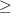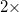trans_lgbasis and trans_rbasistrans_rgbasis must be satisfied. When trans_readhs = 1: 2 Available using the pre-calculated electrode data. 3 The same way as 1 but using the pre-calculated electrode data. This is only for NEGF calculation. RECOMMENDATION:  None Further options are summarized below: trans_printdos (Integer): Controls the printout of TDOS.  0 Default, no total DOS printing. 1 A TDOS (of the junction region) will be printed to TDOS.txt (closed shell). trans_printiv (Integer): Controls printout of calculated current.  0 Default, no current calculated and printed 1 Current will be printed to current.txt (closed shell) or currentA/B.txt (unrestricted or open shell). trans_ipoints (Integer): Number of points for current calculation.  300 Default value. trans_adjustefermi (Integer): Flag to adjust the Fermi energy (FE) for trans_mode = 3 or 4  0 Default, no adjustment. Fixed FE specified by trans_efermi (and trans_efermib) is used. 1 FE is chosen as midpoint of HOMO and LUMO levels 2 FE is adjusted so that charge neutrality is satisfied 3 FE is adjusted by combination way of 1 and 2, i.e. use 2 if maximum difference of density matrix in the iteration is over 10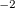and use 3 below that. Options 1, 2, and 3 use the same FE forand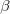spins. -1, -2, -3 are the same as 1, 2, 3, respectively, but allow for different FEs forandspins. trans_nvbias (Integer): (Only for trans_mode = 4), number of points of bias voltage.  1 Default The bias voltage values to be calculated are defined by dividing the range between trans_vstart and trans_vmax with this number. For example, when trans_vstart = 0.0, trans_vmax = 1.0, and trans_nvbias = 5, the voltages are 0.0, 0.25, 0.50, 0.75, and 1.0. For the case of trans_nvbias = 1, the voltage to be calculated is trans_vmax. trans_updatedmatlr (Integer): (Only for trans_mode = 3), flag to update L, R, LC, RC blocks (i.e. except for center block) of density matrix during SCF with zero bias. This is to prepare consistent density matrix with modified Hamiltonian matrix forced by read-in bulk electrode data. Note that it may make it difficult or slow to converge.  0 No update. 1 Update every iteration step (default). 2 Mix the new density matrix with ratio of trans_mixing. The following are parameters are set to a double precision value. The allowed values are set using trans_itodfac as follows: trans_itodfac (Integer): Controls the accuracy for input parameters.  100 Default, the numbers can be of 0.0x precision. 1000 The input double numbers can be of 0.00x precision, etc. trans_vstart (Double): (Only for trans_mode = 4). Starting voltage bias (V). The bias voltage increases from trans_vstart to trans_vmax in the NEGF calculation.  0 Default trans_devsmear (Double): Imaginary smearing (in eV) added to the real Hamiltonian in central region retarded GF evaluation.  0.01 Default, cannot be smaller than 1/trans_itodfac trans_bulksmear (Double): Imaginary smearing (in eV) added to the real Hamiltonian in electrodes GF evaluation.  0.01 Default, cannot be smaller than 1/trans_itodfac trans_greens (Double): Imaginary smearing/Broadening (in eV) added to the Green’s function.  0.07 Default, cannot be smaller than 1/trans_itodfac trans_efermi (Double): Fermi energy of the electrode (forspin) (in eV) used for defining energy range in calculating current for T(E).  -5 Default trans_efermib (Double): Fermi energy forspin (in eV). If this is not given, the same value ofspin is used. trans_vmax (Double): Maximum voltage bias (V).  1 Default trans_gridoffset (Double): (Only for trans_mode = 4), Offset distance (in ) to define the grid box region for bias potential energy.  5 Default The box size is defined by adding the offset distance with maximum and minimum,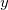andatomic coordinates for each direction. The bias voltage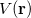on the grid points in the box is used for calculating correction term for the Fock matrix (i.e.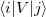). Note that this box is not for correcting electrostatic potential by solving Poisson equation. The grid box region and its grid size for the Poisson equation is given by$plots block keyword (see also example later). The same grid size is used for both boxes.

The following are parameters for when trans_mode = 3, 4:

trans_mixing (Double):
Mixing ratio of DIIS mixing method for updating the central block of density matrix in the NEGF iteration.

 1 Default

trans_mixhistory (Integer):
The number of NEGF iteration steps in which the history of density matrix is stocked for the DIIS method.

 40 Default

trans_dehcir (Double):
Grid size, dE (in eV), for integrating the Greens function on the half circle path on imaginary plane.

 1 Default

trans_delpart (Double):
Grid size, dE (in eV), for integrating the Greens function on the path of the linear part on imaginary plane.

 0.01 Default

trans_debwin (Double):
(For trans_mode = 4). Grid size, dE (in eV), for integration on the non-equilibrium term.

 0.01 Default

trans_numres (Integer):
The number of poles at Fermi energy enclosed by closed contour on the imaginary plane.

 100 Default

trans_peconv (Integer):
The convergence criteria of the iteration of the Poisson equation. The threshold is 10hartree of maximum energy difference over the all grid points.

 9 Default

trans_pemaxite (Integer):
Maximum iteration number of the Poisson equation.

 1000 Default

Flag of read-in electrostatic potential energy. The data is printed in "ReadInESP/" directory with option of -1 or 0, and read from the same directory name with option of 0 or 1.

 -1 Print the read-in ESP data in "ReadInESP/" directory at the first step and stop calculation. 0 Print the read-in ESP data in "ReadInESP/" at the first step and continue calculation using it (default). 1 Read the pre-calculated read-in ESP data from "ReadInESP/" and continue calculation.

trans_restart (Integer):
Flag to restart reading the density matrix files, DAmat.dat (and DBmat.dat) from the "TransRestart/" directory.

 0 No restart (default). 1 Read file of density matrix.

Note: The default energy window for transmission and current calculations is defined as:
trans_emin = trans_efermi - trans_vmax/2 and trans_emax = trans_efermi + trans_vmax/2
if trans_emin and trans_emax are not given.

The trans_emin and trans_emax values can be set to determine the energy window for calculating the transmission function. If specified, these values will override the window defined by trans_efermi and trans_vmax values.

If higher accuracy parameters are set, trans_itodfac must be increased. For example for three digits accuracy (e.g. trans_efermi = -5.341), then trans_itodfac = 1000 or higher must be used. (This parameter applies to all double precision parameters.)

For trans_readhs = 1, the following parameters to parse precalculated Hamiltonian and overlap matrices have to be provided:

• trans_totorb2 (Integer):
Total number of basis functions in the electrode models (if set, then same size is assumed for both electrodes) (no default value).

• trans_totorb2l: Integer number, total number of basis functions in left electrode model (no default value).

• trans_totorb2r: Integer number, total number of basis functions in right electrode model (no default value).

• trans_startpoint: Integer number, start point (basis number) for reading the TB integrals. Note, the basis number, that is, index number of basis function starts from 0. (if set, then same size is assumed for both electrodes) (default value 0).

• trans_startpointl: given as integer number, left start point (basis number) for reading the TB integrals. Note, the basis number, that is, index number of basis function starts from 0. (default value 0).

• trans_startpointr: given as integer number, right start point (basis number) for reading the TB integrals. Note, the basis number, that is, index number of basis function starts from 0. (no default value).

As an example of the Landauer calculation, the sample Q-Chem input is given below.

Example 10.263  Quantum transport Landauer calculation applied to Cbetween two gold electrodes.

$molecule 0 1 Ag -11.0 0 0 Au -8.3 0 0 Ag -5.6 0 0 Au -2.9 0 0 Ag -0.2 0 0 Au 2.5 0 0 C 4.8 0 0 C 6.5 0 0 C 8.2 0 0 C 9.9 0 0 C 11.6 0 0 C 13.3 0 0 Au 15.6 0 0 Ag 18.3 0 0 Au 21.0 0 0 Ag 23.7 0 0 Au 26.4 0 0 Ag 29.1 0 0$end

$rem METHOD B3LYP BASIS lanl2dz ECP lanl2dz GEOM_OPT_MAXCYC 200 INCDFT FALSE MEM_STATIC 8000 MAX_SCF_CYCLES 400 MEM_TOTAL 32000 MOLDEN_FORMAT TRUE SCF_CONVERGENCE 10 SCF_ALGORITHM diis TRANS_ENABLE 1$end

$trans-method trans_spin 0 trans_npoints 300 trans_method 0 trans_readhs 0 trans_printdos 1 trans_efermi -6.50 trans_vmax 4.00$end

$trans-model trans_lgatom 3 trans_latom 5 trans_ratom 14 trans_rgatom 16$end


A sample for unrestricted spin calculation can be found in the $QC/samples/tchem directory. For NEGF calculations, note the followings concerning input etc: • SCF_ALGORITHM = NEGF in$rem is necessary for NEGF calculation.

• The same numbers for trans_lgbasis and trans_lbasis, and also for trans_rgbasis and trans_rbasis must be used.

• Only WBL method for evaluating self-energy is available for the NEGF in the current version (trans_method = 0)

• trans_htype = 3 and trans_readhs = 1 are required

• SYM_IGNORE = TRUE to keep the original input coordinates.

• MEM_TOTAL and MEM_STATIC may need to be set to ensure enough memory is available.

• The bias voltage ofis added on the left electrode, and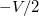on the right electrode, where the bias potential slope is along-axis, i.e. the system structure must be built alongdirection.

• In the $plots section (when defining a grid box for the Poisson equation solving), the grid box region must cover all atoms except for the left and right electrode parts defined in$trans_model. All integer index flags in $plots can be 0. • For calculations on bulk electrode, the same structure with the same orientation in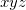-Cartesian coordinate system (but different size) must be used so as to reproduce the same overlap matrix at the used part. • For better calculations, update of electrode relevant (non-center) blocks in the density matrix (trans_updatedmatlr = 1) and Fermi energy to satisfy charge neutrality (trans_adjustefermi = 2 or 3) are recommended at zero bias (trans_opt = 3) before performing a full NEGF calculation. However, these options may make it difficult or slow to reach convergence. • The criterion for convergence in the NEGF iterations is the maximum difference in density matrix elements, and is not a energy threshold. NEGF calculations depend on the following pre-calculated properties: Step 1: Pre-calculations: A: Hamiltonian and overlap matrices for the left and right bulk electrodes (required) B: A converged junction electronic state by standard DFT (recommended) C: Electrostatic potential of large electrode region (optional) Step 2: Self-consistent Greens function calculation with zero bias: Non zero bias cases should be calculated using density matrices calculated with zero bias voltage to obtain converged density matrix. It is also recommend to evaluate the Fermi energy level within the NEGF scheme. Step 3: NEGF calculations should be obtained by increasing the bias sequentially. Examples of these steps applied to Cbetween two aluminium electrodes are given below. Example 10.264 Step 1-A of the NEGF calculation, the pre-calculation of the bulk electrode. Flag keyword of printing matrices must be set (i.e. trans_enable != 0) $molecule
0 1
Al    -15.04250    0.0   0.0
Al    -12.30750    0.0   0.0
Al    -9.572500    0.0   0.0
Al    -6.837500    0.0   0.0
Al    -4.102500    0.0   0.0
Al    -1.367500    0.0   0.0
Al     1.367500    0.0   0.0
Al     4.102500    0.0   0.0
Al     6.837500    0.0   0.0
Al     9.572500    0.0   0.0
Al     12.30750    0.0   0.0
Al     15.04250    0.0   0.0
$end$rem
UNRESTRICTED        true
SYM_IGNORE          true
MAX_SCF_CYCLES       500
EXCHANGE              hf
CORRELATION         none
ECP                 hwmb
BASIS               hwmb
SCF_CONVERGENCE        4
$end @@@$molecule
$end$rem
UNRESTRICTED        true
SYM_IGNORE          true
MAX_SCF_CYCLES       500
EXCHANGE           b3lyp
CORRELATION         none
ECP                 hwmb
BASIS               hwmb
SCF_GUESS_MIX          3
SCF_CONVERGENCE        4
$end @@@$molecule
$end$rem
UNRESTRICTED        true
SYM_IGNORE          true
MAX_SCF_CYCLES       500
EXCHANGE           b3lyp
CORRELATION         none
ECP                 hwmb
BASIS               hwmb
SCF_GUESS_MIX          3
SCF_CONVERGENCE        7
TRANS_ENABLE          -1
$end$trans-method
trans_spin             2
$end  Example 10.265 Step 1-B of the NEGF calculation - the pre-calculation by the standard DFT. The same molecular structure with the step 2 must be used $molecule
0 1
Al  -13.615   0.0   0.0
Al  -10.880   0.0   0.0
Al   -8.145   0.0   0.0
Al   -5.410   0.0   0.0
Al   -2.675   0.0   0.0
C    -0.627   0.0   0.0
C     0.627   0.0   0.0
Al    2.675   0.0   0.0
Al    5.410   0.0   0.0
Al    8.145   0.0   0.0
Al   10.880   0.0   0.0
Al   13.615   0.0   0.0
$end$rem
UNRESTRICTED          true
SYM_IGNORE            true
MAX_SCF_CYCLES        400
EXCHANGE              b3lyp
CORRELATION           none
BASIS                 hwmb
ECP                   hwmb
SCF_CONVERGENCE       5
$end @@@$molecule
$end$rem
UNRESTRICTED          true
SYM_IGNORE            true
EXCHANGE              b3lyp
CORRELATION           none
BASIS                 hwmb
ECP                   hwmb
MAX_SCF_CYCLES        400
SCF_CONVERGENCE       6
SCF_GUESS_MIX         3
$end  Example 10.266 Step 2 of the NEGF calculation. As the preparation of this step, followings are necessary: 1. FAmat2l.dat, FAmat2r.dat, Smat2l.dat, and Smat2r.dat (also FBmat2l.dat and FBmat2r.dat if the calculation is spin-unrestricted ) must be placed in the same directory of the Q-Chem input file by coping or linking the output files of the step 1-A. 2. Restart directory of the standard DFT obtained in the step 1-B must be copied to here. 3. (Optional) Read-in electrostatic potential data in "ReadInESP/" directory must be placed if this option is used (for trans_readesp = 1). This option can provide more bulk electrode electrostatic environment as the boundary condition of Poisson equation solving ( see the sample files in$QC/samples/tchem/) for more details).

$molecule read$end

$rem JOBTYPE sp UNRESTRICTED true SYM_IGNORE true MAXSCF 500 EXCHANGE b3lyp CORRELATION none BASIS hwmb ECP hwmb SCF_CONVERGENCE 4 SCF_ALGORITHM negf SCF_GUESS read MEM_TOTAL 16000 MEM_STATIC 4000 TRANS_ENABLE 1$end

$plots For NEGF (for Poisson equation) 190 -9.5 9.5 80 -4.0 4.0 80 -4.0 4.0 0 0 0 0 0$end

$trans-method trans_opt 3 trans_spin 2 trans_npoints 500 trans_method 0 trans_printdos 1 trans_printiv 1 trans_adjustefermi 1 trans_vmax 1.0 trans_emin -6.5 trans_emax -2.5 trans_mixing 0.1 trans_mixhistory 50 trans_dehcir 1.0 trans_delpart 0.01 trans_numres 100 trans_peconv 8 trans_pemaxite 1000 trans_updatedmatlr 0 trans_readesp 0 trans_htype 3 trans_readhs 1 trans_totorb2 48 trans_startpointl 16 trans_startpointr 32$end

$trans-model trans_lbasis 8 trans_rbasis 8 trans_lgbasis 8 trans_rgbasis 8$end


Example 10.267  Step 3 of the NEGF calculation. As the preparation of this step, followings are necessary:

1. In the same way as step 2, FAmat2l.dat, FAmat2r.dat, Smat2l.dat, and Smat2r.dat (also FBmat2l.dat and FBmat2r.dat for spin-unrestricted calculations) must be placed.

2. Restart directory for Q-Chem generated in the step 2 must be copied to here (only coordinates are used).

3. Restart directory for density matrix "TransRestart/" must be created and DAmat.dat (and DBmat.dat for spin-unrestricted) generated in the step 2 must be copied or linked in the directory.

4. Read-in electrostatic potential data in "ReadInESP/" directory used in the step 2 must be copied to here.

5. Put Fermi energy obtained in Step 2 (recommended).

$molecule read$end

$rem UNRESTRICTED true MAXSCF 500 SYM_IGNORE true EXCHANGE b3lyp ECP hwmb SCF_CONVERGENCE 4 SCF_ALGORITHM negf MEM_TOTAL 16000 MEM_STATIC 4000 TRANS_ENABLE 1$end

$plots For NEGF calculation 190 -9.5 9.5 80 -4.0 4.0 80 -4.0 4.0 0 0 0 0 0$end

$trans-method trans_opt 4 trans_spin 2 trans_npoints 500 trans_method 0 trans_printdos 1 trans_printiv 1 trans_adjustefermi 0 trans_efermi -4.421836 trans_vmax 0.5 trans_emin -6.5 trans_emax -2.5 trans_mixing 0.2 trans_mixhistory 50 trans_dehcir 1.0 trans_delpart 0.01 trans_debwin 0.01 trans_numres 100 trans_peconv 8 trans_pemaxite 1000 trans_gridoffset 4.0 trans_updatedmatlr 0 trans_nvbias 6 trans_restart 1 trans_readesp 1 trans_htype 3 trans_readhs 1 trans_totorb2 48 trans_startpointl 16 trans_startpointr 32$end

$trans-model trans_lbasis 8 trans_rbasis 8 trans_lgbasis 8 trans_rgbasis 8$end